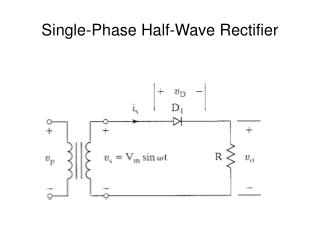DownloadDownload PresentationSingle-Phase Half-Wave Rectifier

# Single-Phase Half-Wave Rectifier

Download Presentation## Single-Phase Half-Wave Rectifier

- - - - - - - - - - - - - - - - - - - - - - - - - - - E N D - - - - - - - - - - - - - - - - - - - - - - - - - - -
##### Presentation Transcript

1. Single-Phase Half-Wave Rectifier

2. Waveforms

3. Single-Phase Half-Wave Rectifier

4. Performance Parameters • Average value of the output voltage, Vdc • Average value of the output current, Idc • Output dc power, Pdc • Pdc = VdcIdc • rms value of the output voltage, Vrms • Output ac power, Pac • Pac = VrmsIrms

5. Performance Parameters (continued) • Efficiency, η • η = Pdc/Pac • Effective (rms) value of the ac component of the output voltage, Vac • Vac = Vrms2 – Vdc2 • Form factor, FF • FF = Vrms/Vdc • Ripple factor, RF • RF = Vac/Vdc

6. Performance Parameters (continued) • Alternate form for ripple factor • Transformer utilization factor, TUF • TUF = Pdc/VsIs • Vs, Is are rms voltage and current of the transformer secondary

7. Input Voltage and Current

8. Performance Parameters (continued) • Displacement angle, Φ • Displacement Factor, DF • DF = cos(Φ) • Harmonic Factor, HF

9. Performance Parameters (continued) • Power Factor, PF

10. Performance Parameters (continued) • Crest Factor, CF

11. Example 3.1 • Determine η, FF, RF, TUF, PIV of the diode, CF of the input current, input PF.

12. Determine the Average Voltage, Vdc

13. Determine the rms Voltage, Vrms

14. Determine Pdc, Pac, and η

15. Determine FF and RF

16. Determine the TUF

17. Determine the PIV • PIV is the maximum (peak) voltage that appears across the diode when reverse biased. Here, PIV = Vm. - - + PIV +

18. Determine CF

19. Determine PF

20. Summary – Half-Wave Rectifier • RF=121% High • Efficiency = 40.5 Low • TUF = 0.286 Low • 1/TUF = 3.496 • transformer must be 3.496 times larger than when using a pure ac voltage source

21. Half-Wave Rectifier with R-L Load

22. Waveforms of Current and Voltage Conduction period of D1 extends beyond ωt = π

23. Average Output Voltage Increase average voltage and current by making σ = 0

24. Waveforms with Dm installed

25. Application as a Battery Charger Diode conducts for vs > E, starting when Vmsinα = E

26. Waveforms for the Battery Charger Diode turns off when vs < E (at β = π – α) Charging current io = (vs – E)/R io = (Vmsinωt – E)/R for α < ωt < β

27. Single-Phase Full-Wave Rectifier Center-Tapped Transformer

28. Waveforms for the Full-Wave Rectifier

29. Single-Phase Full-Wave Rectifier PIV = 2Vm

30. Full-Wave Bridge Rectifier

31. Waveforms for the Full-Wave Bridge

32. Full-Wave Bridge with Waveforms Conduction pattern D1 – D2 D3 – D4 PIV = Vm